CAT  >  Practice Mock Test for SNAP - 18

# Practice Mock Test for SNAP - 18 - CAT

Test Description

## 60 Questions MCQ Test Mock Test Series for SNAP - Practice Mock Test for SNAP - 18

Practice Mock Test for SNAP - 18 for CAT 2023 is part of Mock Test Series for SNAP preparation. The Practice Mock Test for SNAP - 18 questions and answers have been prepared according to the CAT exam syllabus.The Practice Mock Test for SNAP - 18 MCQs are made for CAT 2023 Exam. Find important definitions, questions, notes, meanings, examples, exercises, MCQs and online tests for Practice Mock Test for SNAP - 18 below.
Solutions of Practice Mock Test for SNAP - 18 questions in English are available as part of our Mock Test Series for SNAP for CAT & Practice Mock Test for SNAP - 18 solutions in Hindi for Mock Test Series for SNAP course. Download more important topics, notes, lectures and mock test series for CAT Exam by signing up for free. Attempt Practice Mock Test for SNAP - 18 | 60 questions in 60 minutes | Mock test for CAT preparation | Free important questions MCQ to study Mock Test Series for SNAP for CAT Exam | Download free PDF with solutions
 1 Crore+ students have signed up on EduRev. Have you?
Practice Mock Test for SNAP - 18 - Question 1

### Directions: Read sentence to find out whether there is any grammatical error in it. The sentences are In three separate parts and each one is labelled (a), (b), (c) and (d). In that case, letter (d) will signify a ‘No error’ response.

Detailed Solution for Practice Mock Test for SNAP - 18 - Question 1
Use ‘the’ before latter.
Practice Mock Test for SNAP - 18 - Question 2

### Directions: Spot the error part of the following sentences.

Detailed Solution for Practice Mock Test for SNAP - 18 - Question 2
Use ‘never’ in place of ever’.
Practice Mock Test for SNAP - 18 - Question 3

### Directions: Find out the error part of the following sentences.

Detailed Solution for Practice Mock Test for SNAP - 18 - Question 3
Use ‘he will have read’, in place of ‘he has read’. An action to be completed at certain future point of time will be in Future Perfect Tense.
Practice Mock Test for SNAP - 18 - Question 4

Directions: Read sentence to find out whether there is any grammatical error in it. The sentences are In three separate parts and each one is labelled (a), (b), (c) and (d). In that case, letter (d) will signify a ‘No error’ response.

Detailed Solution for Practice Mock Test for SNAP - 18 - Question 4
There is “No error.” in given sentence.
Practice Mock Test for SNAP - 18 - Question 5

Directions: Spot the error part of the following sentences.

Detailed Solution for Practice Mock Test for SNAP - 18 - Question 5
There is “No error.” in given sentence.
Practice Mock Test for SNAP - 18 - Question 6

Directions: Choose the word most nearly opposite to the given word.

Tragic

Detailed Solution for Practice Mock Test for SNAP - 18 - Question 6

Meaning: Tragic is something sad, harmful or fatal.

Example: It was a tragic love story.

Antonyms: Blessed, Delightful, Fortunate, Harmless

Practice Mock Test for SNAP - 18 - Question 7

Directions: Choose the word most nearly opposite to the given word.

Detailed Solution for Practice Mock Test for SNAP - 18 - Question 7

Meaning: Traduce is to say mean and untrue things about a person or thing, or to betray.

Example: This is the law that traduces one of our most cherished rights.

Synonyms: Defame, Denigrate, Disgrace, Calumniate, Malign

Antonyms: Compliment, Exalt, Flatter, Honor

Practice Mock Test for SNAP - 18 - Question 8

Directions: Spot the error part of the following sentences.

Bhuvan forgot 1)/ to take 2)/ their pen. 3)/ No error 4)

Detailed Solution for Practice Mock Test for SNAP - 18 - Question 8
Replace ‘their’ with ‘his’.

The correct sentence should be: Bhuvan forgot to take his pen.

Practice Mock Test for SNAP - 18 - Question 9

Directions: In the sentence given below, replace the phrase underlined to make the sentence grammatically correct. If the sentence is correct as it is, mark (d) i.e. “No correction required” as the answer.

Q. Whether I stay in Mumbai or Pune, it is all and one to me.

Detailed Solution for Practice Mock Test for SNAP - 18 - Question 9
Correct form: Whether I stay in Mumbai or Pune, it is all one to me.

The phrase 'all one' is used to indicate that it is not important which of several alternatives are available or chosen.

Here, any choice i.e. Mumbai or Pune makes no difference to the person.

Example in a sentence: The priest said, “ Rich and poor are all one to me”.

Practice Mock Test for SNAP - 18 - Question 10

Directions: In the following question, an idiom or proverb has been underlined. Choose its correct meaning in the given context from the alternatives given below.

Q. Her fame spread far and wide because she was a talented singer.

Detailed Solution for Practice Mock Test for SNAP - 18 - Question 10
(a) is the right choice as the fame grows as a result of positive performances and talent.
Practice Mock Test for SNAP - 18 - Question 11

Directions: Choose the word or group of words which is most similar in meaning to the word.

Garrulity

Detailed Solution for Practice Mock Test for SNAP - 18 - Question 11
Meaning: The use of too many words to express an idea.

Synonyms: Circumlocution, Diffuseness, Verbiage

Antonyms: Brevity, Briefness, Terseness

Practice Mock Test for SNAP - 18 - Question 12

Directions: Choose the word or group of words which is most similar in meaning to the word.

Vindicate

Detailed Solution for Practice Mock Test for SNAP - 18 - Question 12

Meaning: To vindicate is to free from a charge of wrongdoing.

Synonyms: Acquit, Clear, Exonerate

Antonyms: Criminate, Incriminate, Accuse

Practice Mock Test for SNAP - 18 - Question 13

Directions: In the following questions, write a single word which can be replaced with the key sentence.

Q. 'A large enclosure for keeping birds' is also called as __________

Detailed Solution for Practice Mock Test for SNAP - 18 - Question 13
The meanings of the words are

Zoo - A large enclosure for wild animals

Aquarium - A large enclosure for fish

Aviary - A large enclosure for keeping birds

Homicide - Killing of human beings

Practice Mock Test for SNAP - 18 - Question 14

Directions: In the following questions, write a single word which can be replaced with the key sentence.

Q. A house where an Eskimo lives.

Detailed Solution for Practice Mock Test for SNAP - 18 - Question 14
Igloo is the right term. Hamlet is another word for village. (d) is the correct answer
Practice Mock Test for SNAP - 18 - Question 15

Find the odd term from the given 4 options which does not belong to the group of remaining options.

Detailed Solution for Practice Mock Test for SNAP - 18 - Question 15
The correct option is (d) Kiwi.

Vulture, Doves and Kingfishers are the birds which have wings and can fly.

Pigeons or doves belong to the bird family Columbidae.

Smaller species are called as 'Dove' and larger ones are pigeons.

Kiwi is a flightless bird. Latin species name for “Kiwi' is Apteryx which means wingless.

Practice Mock Test for SNAP - 18 - Question 16

Four of the following five are alike in a certain way and so form a group. Which is the one that does not belong to that group?

Detailed Solution for Practice Mock Test for SNAP - 18 - Question 16
Except 78, all others give a prime number when 1 is subtracted from them.

78 – 1 = 77

54 – 1 = 53

48 – 1 = 47

42 – 1 = 41

72 – 1 = 71

Practice Mock Test for SNAP - 18 - Question 17

Directions: In these question, relationship between different elements is shown in the statements. The statements are followed by conclusions. Study the conclusions based on the given statements and select the appropriate answer.

Statements: C > W ≥ E = R < B < S: H< R ≥ D

Conculsions

I. C > D

II. H < S

Detailed Solution for Practice Mock Test for SNAP - 18 - Question 17
Statements C > W ≥ E = R < B < S: H< R ≥ D

Therefore, C > W ≥ E = R < B < S IV D

Conclusions

I. C > D(True)

II.H < S(True)

So, both conclusions I and II are true.

Practice Mock Test for SNAP - 18 - Question 18

How many such pairs of letters are there in the word QUICKLY, each of which has as many letters between them in the word (in both forward and backward directions) as in the English alphabetical series?

Detailed Solution for Practice Mock Test for SNAP - 18 - Question 18
Here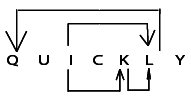Hence, there are four such pairs of letters – IK, IL, KL and LQ.

Practice Mock Test for SNAP - 18 - Question 19

Directions: Study the arrangement carefully and answer the questions given below.

​4 2 6 1 3 8 9 5 8 1 6 5 1 5 8 5 3 9 4 9 2 3 2 1 5 7 2 6 2 4 2 7 4

Q. How many such 2s are there in the above arrangement, each of which is immediately preceded by an odd and also immediately followed by an odd digit?

Detailed Solution for Practice Mock Test for SNAP - 18 - Question 19
According to the question, the arrangements are as shown below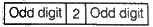923, 321

Note : Some books do not count 1 as an odd number but the concept of odd number is (2 X number + 1). So, if we put 0 at number, then 2 X 0 + 1 = 1, then 1 will be odd number. We all agree that 3 is an odd number how? 2 X 1 + 1 = 3

Practice Mock Test for SNAP - 18 - Question 20

Directions: Ten people are sitting in two parallel rows containing five people each, in such a way that there is equal distance between adjacent persons. In row-1, J, K, L, M and N are seated (not necessarily in the same order) and all of them are facing south. In row-2, V, W, X, Y and Z are seated (not necessarily in the same order) and all of them are facing north. Therefore in the given seating arrangement each member seated in a row faces another member of the other row. Z sits third to the right of W. V sits second to the left of Z. the persons facing V sits to the immediate right of K. Only one person sits between K and M. J is not an immediate neighbour of K. only to people sit between J and L. neither K nor J faces Y

Q. What is the position of Z with respect to Y?

Detailed Solution for Practice Mock Test for SNAP - 18 - Question 20
According to the given information, the sitting arrangement of ten people in two parallel rows is as following

In row-1 J, K, L, M, and N → facing South

In row-2 V, W, X, Y, and Z → facing North

1) Z sits third to the right of W.

2) V sits second to the left of Z.

3) The person facing V sits to the immediate right of K.

4) Only one person sits between K and M.

5) J is not an immediate neighbor of K.

6) Only two people sit between J and L → Case 2 gets eliminated

(If we take 2 persons between J and L, then the place of L will not be determined as it is already occupied by K)

7) Neither K nor J faces Y → Case 1.1 gets eliminated

So, the final arrangement will be

Hence, Z sits to the immediate left of Y.

Practice Mock Test for SNAP - 18 - Question 21

Directions: Seven friends namely A, B, C, D, E, F and G are standing in a straight line facing North but not necessarily in the same order. D stands exactly in the centre of the line. Only two persons stand between D and F.

A stands to the immediate left of C.

A stands to the right of D (may or may not be the immediate right). Only one person stands between A and B. G stands second to the left of B.

Q. Which of the following is true regarding E?

Detailed Solution for Practice Mock Test for SNAP - 18 - Question 21
According to the information, the arrangement of seven friends standing in a straight line is as follows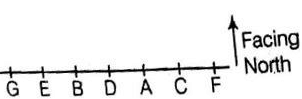Practice Mock Test for SNAP - 18 - Question 22

Directions: Following are the steps of an input. Rearrange them and answer the question.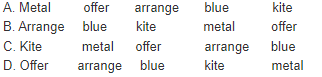Detailed Solution for Practice Mock Test for SNAP - 18 - Question 22
The steps of the input are given in the jumbled form, we have to arrange them properly and answer the following questions.

In the jumbled steps, we notice that the words are in English alphabetical order from the right.

E → Step I kite metal blue offer arrange

C→ Step II kite metal offer arrange blue

A → Step III metal offer arrange blue kite

D → Step IV offer arrange blue kite metal

B → Step V arrange blue kite metal offer

The input designated as (D) is the fourth step

Practice Mock Test for SNAP - 18 - Question 23

Directions: Study the given information and answer the questions.

A number arrangement machine, when given an input line of number rearranges their following a particular rule in each step. The following is the illustration of the input and steps of arrangement. (All the numbers are two-digit number)

Input prepare 14 21 a new method 72 38 97 for studies 68

Step I for a prepare 14 21 new method 72 38 97 studies 68

Step II 21 14 for a prepare new method 72 38 97 studies 68

Step III new method 21 14 for a prepare 72 38 97 studies 68

Step IV 68 38 new method 21 14 for a prepare 72 97 studies

Step V studies prepare 68 38 new method 21 14 for a 72 97

Step VI 97 72 studies prepare 68 38 new method 21 14 for a Step VI is the last step of above arrangement as the intended arrangement is obtained. As per the rules followed in the given steps, find out the appropriate steps for the given input.Input developing 44 markets 38 27 economic practice required 16 83 72 today

Q. Which step are the elements ’72 today required 44’ found in the same order?

Detailed Solution for Practice Mock Test for SNAP - 18 - Question 23
input I developing 44 markets 38 27 practice required 16 83 72 today

Step II27 16 economic developing 44 markets 38 practice required 83 72 today

Step IIIpractice markets 27 16 economic developing 44 38 required 83 72 today

Step IV44 38 practice markets 27 16 economic developing required 83 72 today

Step Vtoday required 44 38 practice markets 27 16 economic developing 83 72

Step VI83 72 today required 44 38 practice markets 27 16 economic developing

There is no such step that matches with the given elements.

Practice Mock Test for SNAP - 18 - Question 24

Directions: The question below consist of a question and two statements numbered I and II given below it. You have to decide whether the data given in the statements are sufficient to answer the questions. Read both the statements and give answer

Q. How is the word ‘GATES’ coded in the code language?

I. ‘BRICK’ is coded as ‘LDJSC’ and ‘PIN’ is coded as ‘OJQ’.

II. ‘WATER’ is coded as ‘SFUBX’ and ‘DISH’ is coded as ‘ITJE’

Detailed Solution for Practice Mock Test for SNAP - 18 - Question 24
The correct option is D The data either in statement I alone or in statement II alone are sufficient to answer the question.

BRICK

First, reverse the word BRICK = KCIRB.

The next alphabet of every letter in the reversed word is LDJSC

The statement II is also folowing same coding pattern as statement I.

So, In the same manner, GATES is coded as:

The reverse of word GATES = SETAG.

The next alphabet of every letter in the reversed word is TFUBH.

So, 'GATES' is coded as 'TFUBH'

So, the data either in statement I alone or in statement II alone are sufficient to answer the question.

Practice Mock Test for SNAP - 18 - Question 25

Directions: Study the following information and answer the question.

Seven people P, Q, R, S, T, U and V have a seminar but not necessarily in the order, on seven different months (of the same year) namely January, February, March, June, August, October and December. Each of them also likes a different fruit namely Banana, Grapes, Papaya, Orange, Mango, Litchi, and Apple but not necessarily in the same order. R has a seminar in a month which has less than 31 days. Only two people have seminar between R and S. The one who likes Banana has a seminar immediately before S. Only one person has a seminar before the one who likes Papaya. Q has a seminar immediately after the one who likes Papaya. Only three people have a seminar between Q and the one who likes Mango.T likes neither Mango nor Papaya. P has a seminar immediately before T. V likes Apple. The one who likes Grapes has a seminar in the month, which has less than 31 days. The one who has a seminar in March does not like Orange.

Q. As per the given arrangement, R is related to Banana and Q is related to Orange following a certain pattern, which of the following is V related to following the same pattern?

Detailed Solution for Practice Mock Test for SNAP - 18 - Question 25
V is related to Litchi.According to the information, the arrangement is as following

 Person Month Fruit V January Apple U February Papaya Q March Litchi R June Grapes P August Banana T October Orange S December Mango

Practice Mock Test for SNAP - 18 - Question 26

Directions: In this question two/three statements followed by two conclusions numbered I and II have been given. You have to take the two/three given statements to be true even if they seem to be at variance from commonly known facts and then decide which of the given conclusions logically follow from the given statements disregarding commonly known facts.

Statements: Some strikes are hits. No strike is a raid. All attacks are raids.

Conclusions

I. No strike is a raid.

II. All attacks being hits is a possibility.

Detailed Solution for Practice Mock Test for SNAP - 18 - Question 26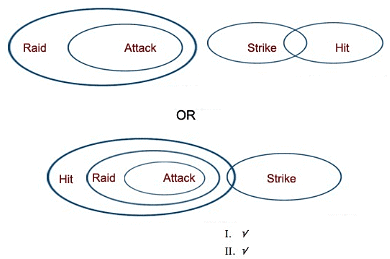So both conclusions I and II are true

Practice Mock Test for SNAP - 18 - Question 27

Directions: In question below a statement is given followed by two courses of action numbered I and II. A course of action is a practicable and feasible step or administrative decision to be taken for follow-up, improvement, or further action in regard to the problem, policy, etc. On the basis of the information given in the statement, you have to assume everything in the statement to be true and decide which of the suggested courses of action logically follow(s) for pursuing.

Statement: There is no motivation among today's generation to join the armed forces owing to frequent transfers to risky areas. Perhaps they are not aware of the good side of it.

Courses of Action

I. Short term internship should be introduced at high school level to give students a peak into the adventurous life of the forces and provide a more realistic job purview.

II. The salary levels of the defense forces should be increased with immediate effect.n.

Detailed Solution for Practice Mock Test for SNAP - 18 - Question 27
For the motivation among today's generation to join armed forces, the right action is that the short term internship should be introduced at High School level to give students a peek into the adventurous life of the Armed Forces. Hence, only action I is the right course of action.
Practice Mock Test for SNAP - 18 - Question 28

Directions: In these question below a statement is given followed by two assumptions/ inferences numbered I and II. An assumption is something supposed or taken for granted and an inference is something which can be directly inferred from the given facts. You have to consider the statement and the following assumptions / inferences and decide which of those is / are implicit in the statement.

Statement: “Travellers with a ticket for the second class, if found travelling in the first class compartments would be penalized''-Notice in the compart- ments of a train. Assumptions

I. Travellers with a ticket for the first class are also not allowed to travel in the second class compartments.

II. Inspections are carried out in the train to check the tickets.

Detailed Solution for Practice Mock Test for SNAP - 18 - Question 28
It is clear that an inspection is carried out and then only such order is given. So, II is implicit in the statement.
Practice Mock Test for SNAP - 18 - Question 29

Directions: In these question below a statement is given followed by two assumptions/ inferences numbered I and II. An assumption is something supposed or taken for granted and an inference is something which can be directly inferred from the given facts. You have to consider the statement and the following assumptions / inferences and decide which of those is / are implicit in the statement.

Statement: Railway does not provide concession to any one for travelling to certain holiday destinations.

Assumptions

I. Railway services are available for travelling to these holiday destinations.

II. Railway provides concession to certain persons for travelling to places other than these holiday destinations.

Detailed Solution for Practice Mock Test for SNAP - 18 - Question 29
It is clear from the statement that the Railway provides concession to certain persons for travelling to places other than these holiday destination. So, assumption II is implicit in the statement.
Practice Mock Test for SNAP - 18 - Question 30

Directions: The questions below are given three statements followed by two conclusions numbered I and II. You have to take the given statements to be true even if they seem to be at variance from commonly known facts.Read all the conclusions and then decide which of the given conclusions logically follows from the given statements disregarding commonly known facts.

Statement: Some ratios are per cent. All per cent are fractions. No fraction is a section.

Conclusions

I. No section is a percent.

II. All ratios being fractions is a possibility.

Detailed Solution for Practice Mock Test for SNAP - 18 - Question 30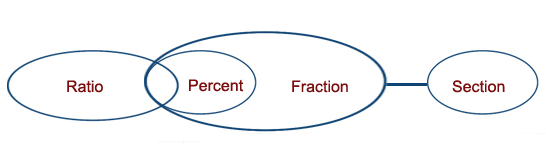So, both conclusion I and II follows.

Practice Mock Test for SNAP - 18 - Question 31

Directions: In this question two/three statements followed by two conclusions numbered I and II have been given. You have to take the two/three given statements to be true even if they seem to be at variance from commonly known facts and then decide which of the given conclusions logically follow from the given statements disregarding commonly known facts.

Statements Some equations are formulae. All equations are terms. All terms are symbols.

Conclusions

I. All equations are symbols

II. No symbol is a formula.

Detailed Solution for Practice Mock Test for SNAP - 18 - Question 31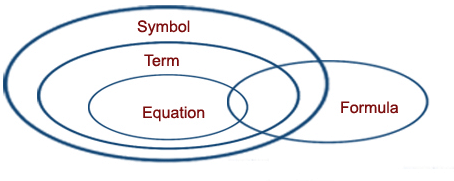So only conclusion I is true.

Practice Mock Test for SNAP - 18 - Question 32

Directions: Study the following information and answer the question.

Seven people P, Q, R, S, T, U and V have a seminar but not necessarily in the order, on seven different months (of the same year) namely January, February, March, June, August, October and December. Each of them also likes a different fruit namely Banana, Grapes, Papaya, Orange, Mango, Litchi, and Apple but not necessarily in the same order. R has a seminar in a month which has less than 31 days. Only two people have seminar between R and S. The one who likes Banana has a seminar immediately before S. Only one person has a seminar before the one who likes Papaya. Q has a seminar immediately after the one who likes Papaya. Only three people have a seminar between Q and the one who likes Mango.T likes neither Mango nor Papaya. P has a seminar immediately before T. V likes Apple. The one who likes Grapes has a seminar in the month, which has less than 31 days. The one who has a seminar in March does not like Orange.

Q. How many people have a seminar between the months in which V and R have a seminar?

Detailed Solution for Practice Mock Test for SNAP - 18 - Question 32
Two people have a seminar between the months in which V and R have a seminar.According to the information, the arrangement is as following
 Person Month Fruit V January Apple U February Papaya Q March Litchi R June Grapes P August Banana T October Orange S December Mango

Practice Mock Test for SNAP - 18 - Question 33

Directions: The question below consist of a question and two statements numbered I and II given below it. You have to decide whether the data given in the statements are sufficient to answer the questions. Read both the statements and give answer.

Q. How many days did Raju take to complete his assignment?

I. Mohit correctly remembers that Raju took more than 3 days but less than 9 days to complete his assignment.

II. Meena correctly remembers that Raju took more than 7 days but less than 11 days to complete his assignment.

Detailed Solution for Practice Mock Test for SNAP - 18 - Question 33
In statement I

According to Mohit, the possible days for Raju to complete his assignment are 4 days, 5 days, 6 days, 7 days or 8 days.

In statement II

According to Meena, the possible days for Raju to complete his assignment are 8 days, 9 days or 10 days.

In statement I and II

8 is common in both the statements. Hence, Raju completes his assignment in 8 days.

Practice Mock Test for SNAP - 18 - Question 34

Directions: Study the given information and answer the question.

A number arrangement machine, when given an input line of number rearranges their following a particular rule in each step. The following is the illustration of the input and steps of arrangement. (All the numbers are two-digit number)

Input prepare 14 21 a new method 72 38 97 for studies 68

Step I for a prepare 14 21 new method 72 38 97 studies 68

Step II 21 14 for a prepare new method 72 38 97 studies 68

Step III new method 21 14 for a prepare 72 38 97 studies 68

Step IV 68 38 new method 21 14 for a prepare 72 97 studies

Step V studies prepare 68 38 new method 21 14 for a 72 97

Step VI 97 72 studies prepare 68 38 new method 21 14 for a Step VI is the last step of above arrangement as the intended arrangement is obtained. As per the rules followed in the given steps, find out the appropriate steps for the given input.Input developing 44 markets 38 27 economic practice required 16 83 72 today

Q. Which of the following is step five of the given input?

Detailed Solution for Practice Mock Test for SNAP - 18 - Question 34
input I developing 44 markets 38 27 practice required 16 83 72 today

Step II27 16 economic developing 44 markets 38 practice required 83 72 today

Step IIIpractice markets 27 16 economic developing 44 38 required 83 72 today

Step IV44 38 practice markets 27 16 economic developing required 83 72 today

Step Vtoday required 44 38 practice markets 27 16 economic developing 83 72

Step VI83 72 today required 44 38 practice markets 27 16 economic developing

Today required 44 38 practice markets 27 16 economic developing 83 27 is the Step V of the given input

Practice Mock Test for SNAP - 18 - Question 35

Directions: Study the following information and answer the given question.

R is married to U. U is the mother of I.L is the sister of D. U has only one daughter. D is married to J. K is the son of J. F is the mother of J.

Q. How is R related to K?

Detailed Solution for Practice Mock Test for SNAP - 18 - Question 35
According to the information, the blood relation can be represented asAs, from the above diagram, R is a male and K is also male. So, R is grandfather to K. Therefore, we cannot determine the relation of R to K from the given options.

Practice Mock Test for SNAP - 18 - Question 36

Directions: Ten people are sitting in two parallel rows containing five people each, in such a way that there is equal distance between adjacent persons. In row-1, J, K, L, M and N are seated (not necessarily in the same order) and all of them are facing south. In row-2, V, W, X, Y and Z are seated (not necessarily in the same order) and all of them are facing north. Therefore in the given seating arrangement each member seated in a row faces another member of the other row. Z sits third to the right of W. V sits second to the left of Z. the persons facing V sits to the immediate right of K. Only one person sits between K and M. J is not an immediate neighbour of K. only to people sit between J and L. neither K nor J faces Y.

Q. Four of the given five are alike in a certain way based on the given arrangement and hence form a group. Which of them does not belong to that group?

Detailed Solution for Practice Mock Test for SNAP - 18 - Question 36
According to the given information, the sitting arrangement of ten people in two parallel rows is as following

In row-1 J, K, L, M, and N → facing South

In row-2 V, W, X, Y, and Z → facing North

1) Z sits third to the right of W.

2) V sits second to the left of Z.

3) The person facing V sits to the immediate right of K.

4) Only one person sits between K and M.

5) J is not an immediate neighbor of K.

6) Only two people sit between J and L → Case 2 gets eliminated

(If we take 2 persons between J and L, then the place of L will not be determined as it is already occupied by K)

7) Neither K nor J faces Y → Case 1.1 gets eliminated

So, the final arrangement will be

Hence, Z sits to the immediate left of Y.

Practice Mock Test for SNAP - 18 - Question 37

Directions: Study the given information carefully to answer the given question

In a certain code language, ‘festival for women only’ is written as ‘ pa ge bo xu’ ‘provide peace to women’ is written as ‘wr dl nj ge’‘women like to celebrate’ is written as ‘ge ct fx wr’ ‘celebrate peace in festival is written as ‘dl bo sv ct

(All codes are two letter codes only)

Q. What is the code for ‘celebrate’ in the given code language?

Detailed Solution for Practice Mock Test for SNAP - 18 - Question 37

The code for ‘celebrate’ in the given code language is ‘ct’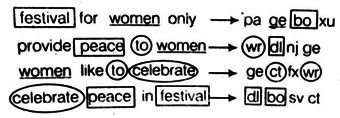The code for ‘festival’ → bo

The code for ‘women’ → ge

The code for ‘peace’ → dl

The code for ‘to’ → wr

The code for ‘celebrate’ → ct

The code for ‘for’ → either ‘pa’ of ‘xu’

The code for ‘only’ → either ‘pa’ of ‘xu’

The code for ‘provide’ → nj

The code for ‘like’ → fx

The code for ‘in’ → sv

Practice Mock Test for SNAP - 18 - Question 38

How many such pairs of letters are there in the word FREQUENT, each of which has as many letters between them in the word (in both forward and backward directions) as in the English alphabetical series?

Detailed Solution for Practice Mock Test for SNAP - 18 - Question 38
Here,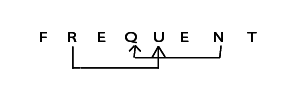Hence, there are two such pairs of letters – RU and QN.

Practice Mock Test for SNAP - 18 - Question 39

Directions: In these questions, relationship between different elements is shown in the statements. The statements are followed by conclusions. Study the conclusions based on the given statements and select the appropriate answer.

Statements

V= D < Y < A ≥ G ≥ E :P= A : R < V

Conclusions

I. E > P

II. P > E

Detailed Solution for Practice Mock Test for SNAP - 18 - Question 39
Statements V = D < Y < A ≥ G ≥ E :P= A ; R < V

Therefore V = D < Y < A ≥ G ≥ E < || P

Conclusions

I. E = P (False)

II. P > E (True)

So only conclusion II is true.

Practice Mock Test for SNAP - 18 - Question 40

Directions: In these question, relationship between different elements is shown in the statements. The statements are followed by conclusions. Study the conclusions based on the given statements and select the appropriate answer.

Statements

S ≤ L≤ I = P E ≥ R : L > Q

Conclusion

I. L > R

II.E ≥ Q

Detailed Solution for Practice Mock Test for SNAP - 18 - Question 40
S ≤ L≤ I = P E ≥ R : L > Q

Therefore,S ≤ L≤ I = P E≥ R Q

Conclusion

I. L> R(Flase)

II.E ≥ Q(Flase)

So, neither conclusion I nor II is true

Practice Mock Test for SNAP - 18 - Question 41

In order that the number 1 y 3 y 6 be divisible by 11, the digit y should be

Detailed Solution for Practice Mock Test for SNAP - 18 - Question 41
The divisibility of 11 says that the difference in the sum of digits at even place to the digits at odd place must be either divisible by 11 or should be 0

Here, the sum of digits at odd place is 10

Sum of digits at even place = 2y

The only possibility is 2y − 10 = 0

⇒ y = 5

Practice Mock Test for SNAP - 18 - Question 42

If the roots x1 and x2 of the quadratic equation x2- 2x + c = 0 also satisfy the equation 7x2 - 4x1 = 47, then which of the following is true?

Detailed Solution for Practice Mock Test for SNAP - 18 - Question 42
7x2 – 4x1 = 47

x1 + x2 = 2

Solving 11x2 = 55

x2 = 5 & x1 = - 3

∴ c = - 15

Practice Mock Test for SNAP - 18 - Question 43

The value of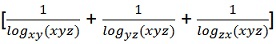is equal to

Detailed Solution for Practice Mock Test for SNAP - 18 - Question 43
Given expression

= logxyz (xy) + logxyz (yz) + logxyz (zx)

= logxyz (xy X yz X zx) = logxyz (xyz)2

= 2 logxyz (xyz) = 2 x 1 = 2

Practice Mock Test for SNAP - 18 - Question 44

If two tangents inclined at an angle of 60º are drawn to a circle of radius 3 cm, then length of each tangent is equal to.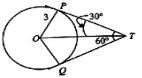Detailed Solution for Practice Mock Test for SNAP - 18 - Question 44

OP / PT = tan30º = 1 / √3

⇒ PT = √3 OP = 3√3

Practice Mock Test for SNAP - 18 - Question 45

The length of a room is double its breadth. The cost of colouring the ceiling at Rs. 25 per sq. m is Rs. 5,000 and the cost of painting the four walls at Rs. 240 per sq. m is Rs. 64,800. Find the height of the room.

Detailed Solution for Practice Mock Test for SNAP - 18 - Question 45
Let the length of the room be l m

Therefore,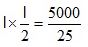Also,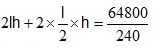⇒ 3lh = 270

Or h =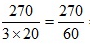= 4.5 cm

Practice Mock Test for SNAP - 18 - Question 46

The number of words from the letters of the words BHARAT in which B and H will never come together, is

Detailed Solution for Practice Mock Test for SNAP - 18 - Question 46
There are 6 letters in the word BHARAT, 2 of them are identical.

Hence total number of words with these letter = 360

Also the number of words in which B and H come together = 120

∴ The required numbers of words = 360 – 120 = 240

Practice Mock Test for SNAP - 18 - Question 47

From eighty cards numbered 1 to 80, two cards are selected randomly. The probability that both the cards have the numbers divisible by 4 is given by.

Detailed Solution for Practice Mock Test for SNAP - 18 - Question 47
Total no. of numbers divisible by 4 between 1 to 80

80 = 4 + (n – 1)4

80 = 4n

⇒ n = 20

∴ Required Probability = 20C2 / 80C2 = 19/316

Practice Mock Test for SNAP - 18 - Question 48

Which of the following relationship is true ?

Detailed Solution for Practice Mock Test for SNAP - 18 - Question 48
sin A/cosA = tan A
Practice Mock Test for SNAP - 18 - Question 49

A factory was opened in 1994 with certain initial strengths in different untis as shown in the table. At the beginning of the subsequent years some of the workers left and some new workers were deployed. No worker left or joined in between. Details are given in the table given below. Study it carefully and answer the questions that follow.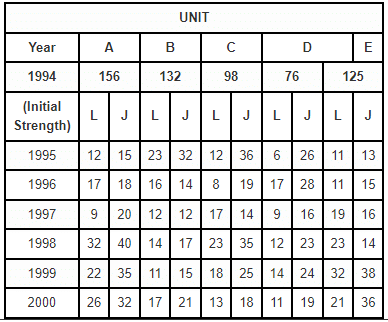Q. 1999 the strength of workers was maxmium in which unit ?

Detailed Solution for Practice Mock Test for SNAP - 18 - Question 49
Strength of worders in 1999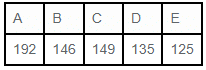Practice Mock Test for SNAP - 18 - Question 50

Directions: Each of the question below consists of a question and two statements numbered (I) and (II) given below it. You have to decide whether the data provided in the statements are sufficient to answer the question.

Q. How Many litres of a chemical can be stored in a cylindrical tank if the radius of the tank is 5 meters?

(I) The height of tank is 5m.

(II) The temperature is 70⁰F.

Detailed Solution for Practice Mock Test for SNAP - 18 - Question 50
Storing capacity of tank = volume of tank = πr2h

Here r is given

And (I) → height (h)

Statement (I) alone is sufficient to answer the question.

(II) →There is no need of value of temperature to answer the question.

Practice Mock Test for SNAP - 18 - Question 51

Directions: Each of the question below consists of a question and two statements numbered (I) and (II) given below it. You have to decide whether the data provided in the statements are sufficient to answer the question.

Q. What selling price should be marked on the article?

(I) Discount of 5% is to be given and Profit percentage should be double the discount. Purchase cost is in the range of Rs 300 – Rs 400.

(II) 10% discount is to be allowed and 15% profit is to be obtained on the purchase cost of Rs 200 of the article.

Detailed Solution for Practice Mock Test for SNAP - 18 - Question 51
(I) → The fixed value of CP is not given, so, SP of the article cannot be determined

(II) → Let x be SP of an article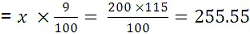Hence, we can find the answer using statement II alone.

Practice Mock Test for SNAP - 18 - Question 52

Percentage of students passed over appeared from six States over the years in an admission test.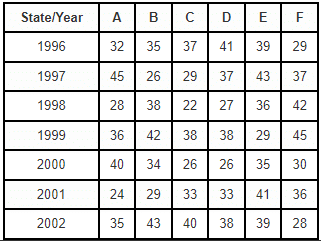Q. If the average number of students qualified from State E for the given years was 532, what was the average number of students appeared?

Detailed Solution for Practice Mock Test for SNAP - 18 - Question 52
Total no. of student qualified from state E over the years = 532 × 7 = 3724.
Practice Mock Test for SNAP - 18 - Question 53

If cos (40° + x) = sin 30°, then x is equal to

Detailed Solution for Practice Mock Test for SNAP - 18 - Question 53
cos (40° + x) = sin 30°

⇒ cos (40° + x) = cos 60°

⇒ 40° + x = 60°

⇒ x = 20°

Practice Mock Test for SNAP - 18 - Question 54

The probability of getting sum more than 7 when a pair of dice are thrown is

Detailed Solution for Practice Mock Test for SNAP - 18 - Question 54
Here n (S) = 62 = 36

Let E be the event “getting sum more than 7” i.e. sum of pair of dice = 8, 9, 10, 11, 12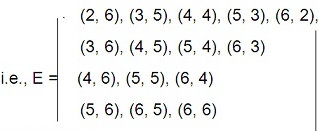∴ n (E) = 15

∴ Required Probability = n (E) / n (S) = 15/36 = 5/12

Practice Mock Test for SNAP - 18 - Question 55

How many different ways are possible to arrange the letters of the word “MACHINE” so that the vowels may occupy only the odd positions?

Detailed Solution for Practice Mock Test for SNAP - 18 - Question 55
MACHINE has 4 consonants and 3 vowels.

The vowels can be placed in position no. 1, 3, 5, 7

→ Total number of ways possible = 4P3 = 24

For each of these 24 ways the 4 consonants can occupy the other 4 places in 4P4 ways

→ Total = 24 x 24 = 576

Practice Mock Test for SNAP - 18 - Question 56

The line x + y = 4 divides the line joining the points (- 1, 1) and (5, 7) in the ratio.

Detailed Solution for Practice Mock Test for SNAP - 18 - Question 56

Ratio = - (-1 + 1 - 4 / 5 + 7 - 4) = 1/2

Practice Mock Test for SNAP - 18 - Question 57

The ratio between the length and the breadth of a rectangular park is 3 : 2 If a man cycling along the boundary of the park at the speed of 12km/hr completes one round it 8 minutes, then the area of the park (in sq. m) is :

Detailed Solution for Practice Mock Test for SNAP - 18 - Question 57
Perimeter = Distance covered in 8 min.

=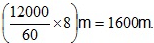Let length = 3x metres and breadth = 2x metres.

Then, 2 (3x + 2x) = 1600 or x = 160.

Length = 480 m and Breadth = 320 m.

Area = (480 × 320)m2 = 153600 m2

Practice Mock Test for SNAP - 18 - Question 58

In the adjoining figure, ABCD is a cyclic quadrilateral. If AB is a diameter, BC = CD and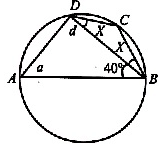Detailed Solution for Practice Mock Test for SNAP - 18 - Question 58
In DBCD, BC = CD,

⇒ 40º + x + 90º + x = 180º

⇒ x = 25º

Practice Mock Test for SNAP - 18 - Question 59

For what value of k are the roots of the quadratic equation (k + 1) x2 – 2 (k – 1) x + 1 = 0 real and equal ?

Detailed Solution for Practice Mock Test for SNAP - 18 - Question 59
Since, the roots of the equation

(k + 1)x2 – 2 (k – 1) x + 1 = 0 are real and equal.

∴ D = 0

∴ {- 2 (k – 1)}2 – 4 (k + 1) = 0 (b2 – 4ac = 0)

⇒ 4 (k2 – 2k + 1) – 4 (k + 1) = 0

⇒ k2 – 2k + 1 – k – 1 = 0

⇒ k2 – 3k = 0

⇒ k = 0, k = 3

Practice Mock Test for SNAP - 18 - Question 60

In a certain party, there was a bowl of rice for every two guests, a bowl of broth for every three of them and a bowl of meat for every four of them. If in all there were 65 bowls of food, then how many guests were there in the party?

Detailed Solution for Practice Mock Test for SNAP - 18 - Question 60
Let the number of rice bowls be x,

The number of broth bowls be y

And the number of meat bowls be z.

Now, x + y + z = 65 …(1)

And 2x = 3y = 4z …(2)

From (1) and (2), we have x = 30, y = 20, z = 15

Thus, the total number of guests = 2x = 3y = 4z = 60

## Mock Test Series for SNAP

16 docs|33 tests
Information about Practice Mock Test for SNAP - 18 Page
In this test you can find the Exam questions for Practice Mock Test for SNAP - 18 solved & explained in the simplest way possible. Besides giving Questions and answers for Practice Mock Test for SNAP - 18, EduRev gives you an ample number of Online tests for practice

## Mock Test Series for SNAP

16 docs|33 tests

### How to Prepare for CAT

Read our guide to prepare for CAT which is created by Toppers & the best Teachers(Scan QR code)